# 2) A person paid \$1,040 for a five year, \$1,000, 8% bond. Required: a) Determine how...

2) A person paid \$1,040 for a five year, \$1,000, 8% bond.

Required:

1. a) Determine how much cash the bondholder will receive each year as interest.

2. b) Determine the amount of cash the bondholder will receive for his return on investment

and the return of his investment for the \$1,000 bond. Be sure to show your work.

3. c) Determine if the effective (market) interest rate is less than, equal to, or greater than

12%.

· [a] Cash to be received each year as interest = \$ 1000 x 8% = \$ 80

· [b]
Amount of cash received by bondholder will include:
>Interest amount of \$ 80 x 5 years = \$ 400
>Investment amount = \$ 1000

· [c]
Effective interest rate is LESS than 12%
This is because Issue price is MORE than face value of Bonds, which states that issuer is charging premium for providing HIGHER stated interest rate, while market rate is below than 8%.

##### Add Answer to: 2) A person paid \$1,040 for a five year, \$1,000, 8% bond. Required: a) Determine how...
Similar Homework Help Questions
• ### 1) A person paid \$950 for a five year, \$1,000, 9% bond. Required: a) Determine how...

1) A person paid \$950 for a five year, \$1,000, 9% bond. Required: a) Determine how much cash the bondholder will receive each year as interest. b) Determine the amount of cash the bondholder will receive for his return on investment (950 paid) and the return of his investment for the \$1,000 bond. Be sure to show your work. c) Determine if the effective (market) interest rate is less than, equal to, or greater than 12%.

• ### At t=0, you purchase a five-year, 8 percent coupon bond (paid annually) that is priced at...

At t=0, you purchase a five-year, 8 percent coupon bond (paid annually) that is priced at par. The face value of the bond is \$1,000. You are also given that your investment horizon is also five years. Suppose that the market interest rate increases to 9 percent (increase by 100 basis points) during the first year of your purchase (within year 1), and it remains at that level (9 percent) for the next four years. You decided to sell the...

• ### QUESTION 4 IBM's bonds currently sell for \$1,040 and have a par value of \$1,000. They...

QUESTION 4 IBM's bonds currently sell for \$1,040 and have a par value of \$1,000. They pay \$65 annual coupon and have a 15 year maturity, but may be called in 5 years at \$1,000. What is their Yield to Maturity (YTM)? 5.78% 6.39% 6.71% 6.09% QUESTION 5 Bob's corporation's bonds make an annual payment of 7.35%. The bonds have a par value of \$1,000, a current price of \$1,130, and mature in 12 years. What is the yield to...

• ### 8. Bond valuation The process of bond valuation is based on the fundamental concept that the...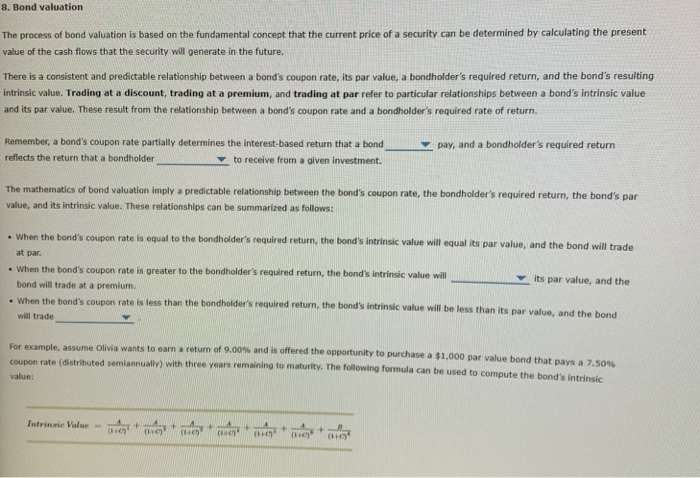8. Bond valuation The process of bond valuation is based on the fundamental concept that the current price of a security can be determined by calculating the present value of the cash flows that the security will generate in the future. There is a consistent and predictable relationship between a bond's coupon rate, its par value, a bondholder's required return, and the bond's resulting intrinsic value. Trading at a discount, trading at a premium, and trading at par refer to...

• ### 2. Bond valuation The process of bond valuation is based on the fundamental concept that the...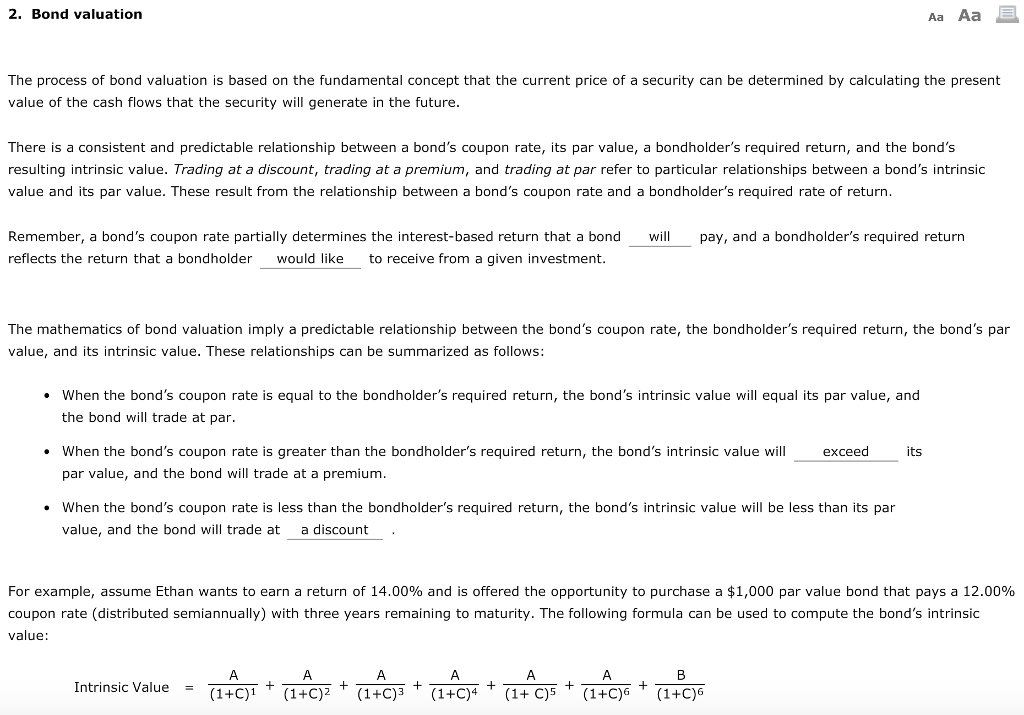2. Bond valuation The process of bond valuation is based on the fundamental concept that the current price of a security can be determined by calculating the present value of the cash flows that the security will generate in the future There is a consistent and predictable relationship between a bond's coupon rate, its par value, a bondholder's required return, and the bond's resulting intrinsic value. Trading at a discount, trading at a premium, and trading at par refer to...

• ### Please determine the correct answers below. When a bond is sold at premium, issue price is...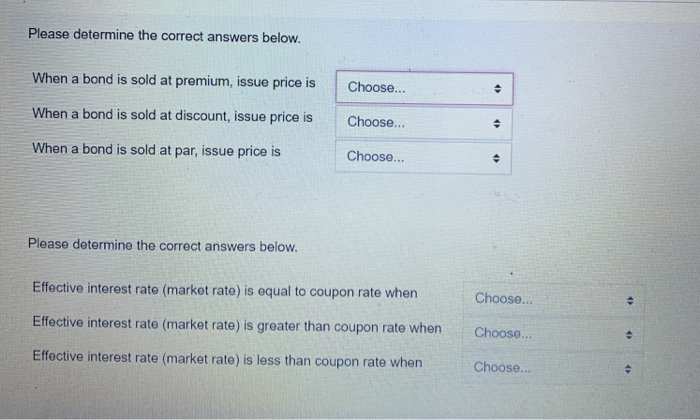Please determine the correct answers below. When a bond is sold at premium, issue price is Choose... . When a bond is sold at discount, issue price is Choose... . When a bond is sold at par, issue price is Choose... 0 Please determine the correct answers below. Effective interest rate (market rate) is equal to coupon rate when Choose... Effective interest rate (market rate) is greater than coupon rate when Choose... . Effective interest rate (market rate) is less...

• ### A 10-year bond that has a 12 percent coupon rate is currently selling for \$1,000, which...

A 10-year bond that has a 12 percent coupon rate is currently selling for \$1,000, which equals the bond's face value. If interest is paid semiannually, the bond's yield to maturity is (a)  equal to 12%. (b)  greater than 12%. (c)  less than 12%. (d)  More information is needed to answer this question. (e) None of the above is correct.

• ### The market price of an 8 year, zero coupon bond is \$476. Your cousin asks you...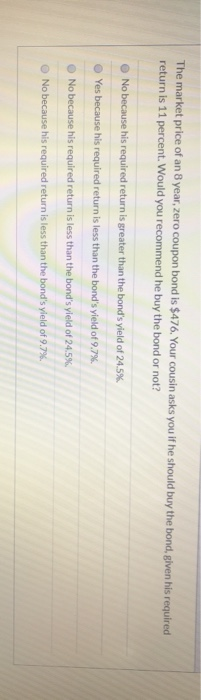The market price of an 8 year, zero coupon bond is \$476. Your cousin asks you if he should buy the bond, given his required return is 11 percent. Would you recommend he buy the bond or not? No because his required return is greater than the bond's yield of 24.5% Yes because his required return is less than the bond's yield of 9.7% No because his required return is less than the bond's yield of 24.5% No because his...

• ### show all work Mr. Bond is considering purchasing a bond with 10-year maturity and \$1,000 face...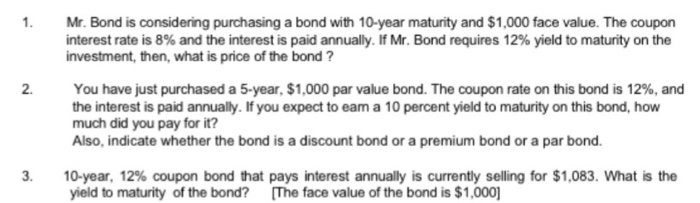show all work Mr. Bond is considering purchasing a bond with 10-year maturity and \$1,000 face value. The coupon interest rate is 8% and the interest is paid annually. If Mr. Bond requires 12% yield to maturity on the investment, then, what is price of the bond ? You have just purchased a 5-year, \$1,000 par value bond. The coupon rate on this bond is 12%, and the interest is paid annually. If you expect to eam a 10 percent...

• ### You are considering a 15-year, \$1,000 par value bond. Its coupon rate is 8%, and interest is paid semiannually. If you r...

You are considering a 15-year, \$1,000 par value bond. Its coupon rate is 8%, and interest is paid semiannually. If you require an "effective" annual interest rate (not a nominal rate) of 8.05%, how much should you be willing to pay for the bond? Do not round intermediate steps. Round your answer to the nearest cent.

Free Homework App